• sympy是python的一个科学计算库，用强大的符号计算体系完成诸如多项式求值，求极限，求导，解放长求积分，解微分方程，级数展开，矩阵运算等功能。 如： 常用的sympy内置符号 自然对数：sympy.E 无穷大：sympy.oo...
SymPy
sympy是python的一个科学计算库，用强大的符号计算体系完成诸如多项式求值，求极限，求导，解放长求积分，解微分方程，级数展开，矩阵运算等功能。
如：
常用的sympy内置符号

自然对数：sympy.E
无穷大：sympy.oo（是字母oo）
圆周率π：sumpy.py

import sympy
import numpy as np
print(np.double(sympy.log(sympy.E*sympy.pi)))

2.1447298858494

用sympy进行初等运算
常用的函数有：

对数函数sympy.log
正弦函数sympy.sin
求平方根函数sympy.sqrt
n次方跟函数sympy.root
求阶乘sympy.factorial

函数求解：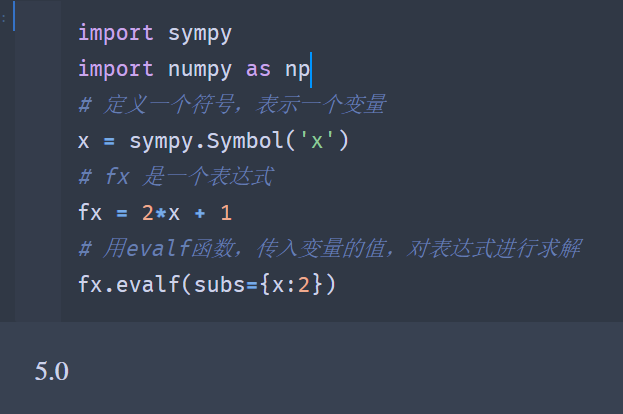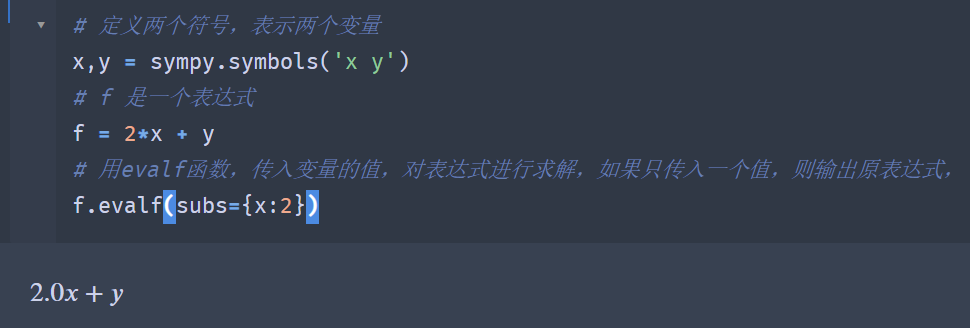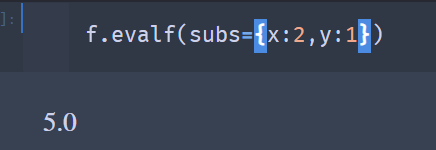函数求极限：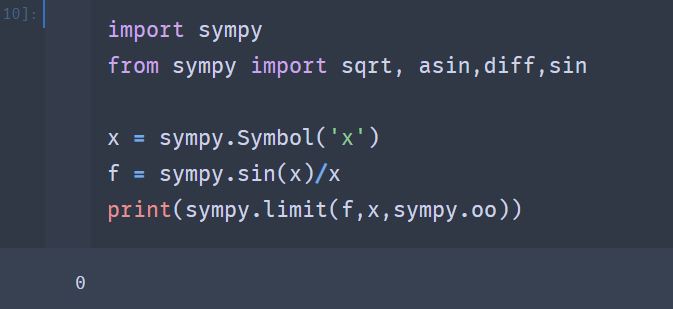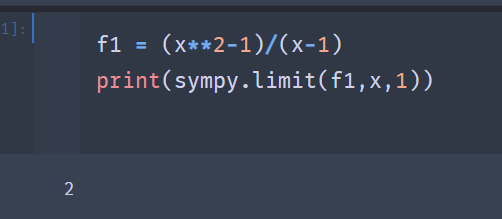函数求导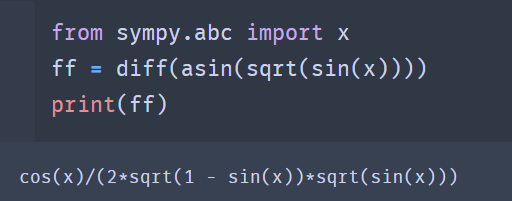NumPy
numpy支持大量的为数组与矩阵运算，此外也针对数运算，提供大量的数学函数库。numpy是一个运行速度非常快的数学库，主要用于数组计算。
numpy通常与scipy和matplotlib一起使用，这种组合广泛用于替代MATLAB，是一个强大的科学计算环境。
很多详细操作在这里有写：这就是数据分析之numpy
SciPy
scipy是著名的科学计算库，构建在numpy之上功能更为强大，scipy函数在numpy的基础上增加了众多的函数，以及工程计算中常用的库函数，可以说，numpy是一个纯数学的计算模块，而cdipy是一个更高阶的科学计算库，
求导
对$x^5$求导
from scipy.misc import derivative
# 定义函数
def f(x):
return x**5
# 对函数在x =2处求导
print(derivative(f,2,dx=1e-6))

对多项式$x^5+2x^4+3x^2+5$求导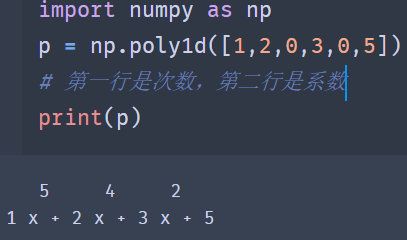求函数的一阶导数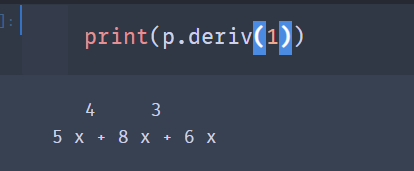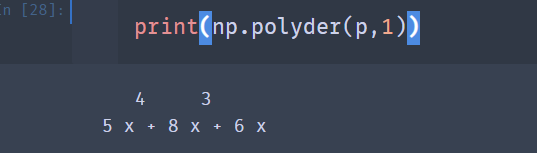求函数的一阶导数在x=1时的值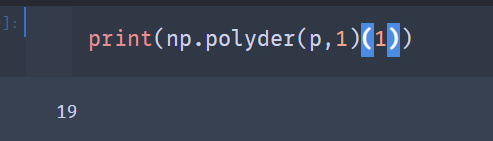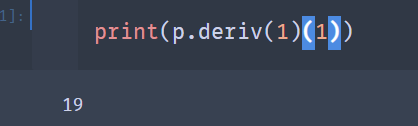求积分
$\int_{0}^{3}\cos^2(e^x)dx$
可以用quad函数，他的一般形式是scipy.integrate.quad(f,a,b)其中f是积分的函数名称，a，b为积分下限和上限。
import numpy as np
f = lambda x:np.cos(np.exp(x))**2
print(solution)

# 前一个为积分求得的值，后一个为误差
(1.296467785724373, 1.397797133112089e-09)

求二重积分(曲线积分)：
$\int\int e^{-x^2-y^2}dxdy$，其中D={(x,y)|0=<x=<10，0=<y=<10}
dblquad函数的一般形式为scipy.integrate.dblquad(func,a,b,gfun,hfun)
其中func是要积分的函数名称，a，b为别是变量x的下限和上限，gfun和hhen是定义变量y的下限和上限的函数名。即使gfun和hfun是常数，他们很多情况下也必须定义为函数。如定义gfun(x)=0，gfun(x)=10，lambda x:y_a和lambda x:y_b，其中y_a，y_b分别为0和10；
import numpy as np
def integrand(x,y):
return np.exp(-x**2-y**2)

x_a = 0
x_b = 10
y_a = 0
y_b = 10

print(solution,abserr)

0.7853981633974476 1.375309851021853e-08

用面积法，自己写个积分函数
$\int_{0}^{3}\cos^2(e^x)dx$
import numpy as np
def fxx(x):
return (np.cos(np.exp(x))) ** 2
n=1000
a = np.linspace(0,3,n)
h = 3/n
sums = 0
for i in a:
sums += fxx(i)*h
print(sums)展开全文numpy 人工智能 机器学习 数据分析
• 一、NumPy简介NumPy系统是Python的一种开源的数值计算扩展。这种工具可用来存储和处理大型矩阵，比Python自身的嵌套列表(nested list structure)结构要高效的多(该结构也可以用来表示矩阵(matrix))。以下为官方英文...一、NumPy简介NumPy系统是Python的一种开源的数值计算扩展。这种工具可用来存储和处理大型矩阵，比Python自身的嵌套列表(nested list structure)结构要高效的多(该结构也可以用来表示矩阵(matrix))。以下为官方英文介绍：NumPy is the fundamental package for scientific computing with Python. It contains among other things:(1)a powerful N-dimensional array object(2)sophisticated (broadcasting) functions(3)tools for integrating C/C++ and Fortran code(4)useful linear algebra, Fourier transform, and random number capabilitiesBesides its obvious scientific uses, NumPy can also be used as an efficient multi-dimensional container of generic data. Arbitrary data-types can be defined. This allows NumPy to seamlessly and speedily integrate with a wide variety of databases.二、python环境注意事项目前python3使用也比较多，有的同学可能安装了两个版本，包括python2，Mac 在终端中输入python命令后，输出的信息还是Python 2.7。如何让系统默认使用python3呢？按照以下步骤设置即可：(1) 终端输入：open ~/.bash_profile，打开.bash_profile文件。(2)修改.bash_profile文件内容 ，添加如下两行PATH="/Library/Frameworks/Python.framework/Versions/3.6/bin:\${PATH}"export PATH(3)添加别名 alias python="/Library/Frameworks/Python.framework/Versions/3.6/bin/python3.6"(4)重新生效.bash_profile文件source .bash_profile经过以上四步，终端输入phtyon，出现以下内容即修改成功。三、NumPy安装配置好python环境后，在命令行输入：from numpy import*，如果提示以下错误，我们需要手动安装NumPy：(1)pip安装我们使用pip安装NumPy，前提是已经安装pip，mac默认是没有安装pip的。如果用的是macOS自带的python2.7，直接终端输入：sudo easy_install pip，安装。如果使用的Python3，终端输入：curl https://bootstrap.pypa.io/get-pip.py | python3。安装完成后，查看pip的版本和路径。由于我分别安装了python2和3，所以也分别安装了pip的不同版本，如下：注意：1)使用pip install XXX新安装的库会放在这个目录下面python2.7/site-packages2)使用pip3 install XXX新安装的库会放在这个目录下面python3.6/site-packages在使用python3执行程序，那么就不能import python2.7/site-packages中的库。(2)安装NumPy终端输入：sudo pip3 install -U numpy安装成功输出以下log：四、NumPy使用(1)基础NumPy的主要对象是同类型的多维数组。它是一张表，所有元素(通常是数字)的类型都相同，并通过正整数元组索引。在NumPy中，维度称为轴。轴的数目为rank。例如，3D空间中的点的坐标[1, 2, 1]是rank为1的数组，因为它具有一个轴。该轴的长度为3。在下图所示的示例中，数组的rank为2(它是2维的)。第一维度(轴)的长度为2，第二维度的长度为3。[[ 1., 0., 0.],[ 0., 1., 2.]]NumPy的数组的类称为ndarray。别名为array。请注意，numpy.array与标准Python库的类array.array不同，后者仅处理一维数组并提供较少的功能。ndarray对象的更重要的属性是：ndarray.ndim数组的轴(维度)的个数。在Python世界中，维度的数量被称为rank。ndarray.shape数组的维度。这是一个整数的元组，表示每个维度中数组的大小。对于具有n行和m列的矩阵，shape将是(n,m)。因此，shape元组的长度就是rank或维度的个数ndim。ndarray.size数组元素的总数。这等于shape的元素的乘积。ndarray.dtype描述数组中元素类型的对象。可以使用标准Python类型创建或指定dtype。另外NumPy提供了自己的类型。例如numpy.int32、numpy.int16和numpy.float64。ndarray.itemsize数组中每个元素的字节大小。例如，元素为float64类型的数组的itemsize为8(=64/8)，而complex32类型的数组的comitemsize为4(=32/8)。它等于ndarray.dtype.itemsize。ndarray.data该缓冲区包含数组的实际元素。通常，我们不需要使用此属性，因为我们将使用索引访问数组中的元素。举例说明：import numpy as np#这里我们生成了一个一维数组a，从0开始，步长为1，长度为20a = np.arange(15)print('a:',a)#打印a的类型，应该是arrayprint('a type:',type(a))#通过函数"reshape"，我们可以重新构造一下这个数组，例如，我们可以构造一个3*5的二维数组a = a.reshape(3, 5)print('a.reshape:',a)#数组的维度print('a.shape:',a.shape)#数组的维度的个数print('a.ndim:',a.ndim)#数组元素总数print('a.size:',a.size)#数组元素类型print('a.dtype:',a.dtype)#数组元素的字节大小print('a.itemsize:',a.itemsize)对应输出如下：a: [ 0  1  2  3  4  5  6  7  8  9 10 11 12 13 14]a type: a.reshape: [[ 0  1  2  3  4][ 5  6  7  8  9][10 11 12 13 14]]a.shape: (3, 5)a.ndim: 2a.size: 15a.dtype: int64a.itemsize: 8(2)数组创建有几种方法来创建数组，可以使用array函数从常规Python列表或元组中创建数组。得到的数组的类型从序列中元素的类型推导出。>>> a = array( [2,3,4] )>>> aarray([2, 3, 4])>>> a.dtypedtype('int32')>>> b = array([1.2, 3.5, 5.1])>>> b.dtypedtype('float64')  一个常见的错误包括用多个数值参数调用array而不是提供一个由数值组成的列表作为一个参数。>>> a = array(1,2,3,4)    # WRONG>>> a = array([1,2,3,4])  # RIGHT(3)数组打印当你打印一个数组，NumPy以类似嵌套列表的形式显示它，但是呈以下布局：最后的轴从左到右打印次后的轴从顶向下打印剩下的轴从顶向下打印，每个切片通过一个空行与下一个隔开一维数组被打印成行，二维数组成矩阵，三维数组成矩阵列表。>>> a = arange(6)                         # 1d array>>> print a[0 1 2 3 4 5]>>>>>> b = arange(12).reshape(4,3)           # 2d array>>> print b[[ 0  1  2][ 3  4  5][ 6  7  8][ 9 10 11]]>>>>>> c = arange(24).reshape(2,3,4)         # 3d array>>> print c[[[ 0  1  2  3][ 4  5  6  7][ 8  9 10 11]][[12 13 14 15][16 17 18 19][20 21 22 23]]](4)数组运算数组上的算术运算符使用元素级别。将创建一个新数组并用结果填充。>>> a = np.array( [20,30,40,50] )>>> b = np.arange( 4 )>>> barray([0, 1, 2, 3])>>> c = a-b>>> carray([20, 29, 38, 47])>>> b**2array([0, 1, 4, 9])>>> 10*np.sin(a)array([ 9.12945251, -9.88031624,  7.4511316 , -2.62374854])>>> a<35array([ True, True, False, False], dtype=bool)与许多矩阵语言不同，乘法运算符*的运算在NumPy数组中是元素级别的。可以使用dot函数或方法执行矩阵乘积：>>> A = np.array( [[1,1],...             [0,1]] )>>> B = np.array( [[2,0],...             [3,4]] )>>> A*B                         # elementwise productarray([[2, 0],[0, 4]])>>> A.dot(B)                    # matrix productarray([[5, 4],[3, 4]])>>> np.dot(A, B)                # another matrix productarray([[5, 4],[3, 4]])某些操作(如+=和*=)可以修改现有数组，而不是创建新数组。>>> a = np.ones((2,3), dtype=int)>>> b = np.random.random((2,3))>>> a *= 3>>> aarray([[3, 3, 3],[3, 3, 3]])>>> b += a>>> barray([[ 3.417022  ,  3.72032449,  3.00011437],[ 3.30233257,  3.14675589,  3.09233859]])>>> a += b                  # b is not automatically converted to integer typeTraceback (most recent call last):...TypeError: Cannot cast ufunc add output from dtype('float64') to dtype('int64') with casting rule 'same_kind'此外，NumPy还有很多强大的功能，大家如果有需要可以到官方参考文档。
展开全文• 以下是自己写的一个python脚本，用来进行高斯展开的，可发现运行速度特别慢，短板应该是数值计算，请问有没有什么方式可以加快它的运行速度？另外，我一直是自学的，代码肯定有许多不...以下是自己写的一个python...
以下是自己写的一个python脚本，用来进行高斯展开的，可发现运行速度特别慢，短板应该是数值计算，请问有没有什么方式可以加快它的运行速度？另外，我一直是自学的，代码肯定有许多不...以下是自己写的一个python脚本，用来进行高斯展开的，可发现运行速度特别慢，短板应该是数值计算，请问有没有什么方式可以加快它的运行速度？另外，我一直是自学的，代码肯定有许多不足之处，还望指点...#!/usr/bin/python3.4import numpy as npimport sysdef gaussian(start=0, end=10, step=0.5, parameter=('sigma', 0.1), inp=[]):if parameter=='sigma':sigma=parameterelif parameter=='FWHM':sigma=parameter/(2*np.sqrt(2*np.log(2)))else:sigma=1print('sigma is set to 1')i=startoutp=[]while i<=end:height=0for j in inp:try:height+=j*np.exp(-(j-i)**2/(2*sigma**2))/(sigma*np.sqrt(2*np.pi))except IndexError:height+=np.exp(-(j-i)**2/(2*sigma**2))/(sigma*np.sqrt(2*np.pi))outp.append((i, height))i+=stepreturn outpf=open(sys.argv, 'r')lines=f.readlines()f.close()inp=[]for i in range(len(lines)):hang=[]for j in lines[i].split():hang.append(float(j))inp.append(hang)outp=gaussian(float(sys.argv), float(sys.argv), float(sys.argv), (sys.argv, float(sys.argv)), inp)text=''for i in range(len(outp)):text+='%15.9f%15.9f\n' % (outp[i], outp[i])w=open('gaussian.dat', 'w')w.write(text)w.close()展开
展开全文• print "Welcome to run" print "Please input num" print "1 stand for 矩形" print "2 stand for 圆" print "3 stand for 正方形" s=int(input("Please input U num:")) ... print "input 长...

print "Welcome to run"
print "1 stand for 矩形"
print "2 stand for 圆"
print "3 stand for 正方形"
if s == 1:
print "input 长度:"
print "input 宽度:"
a=int(input("长度:"))
b=int(input("宽度:"))
x=a*b
print x
elif s == 3:
print "input 正方形边长"
v=int(input("边长:"))
z=v*v
print v
elif s == 2:
print "input 值:"
c=int(input("半径:"))
d=c*c*3.14
print d
else:
print "OH no! Please input 1-3"

转载于:https://blog.51cto.com/ygongzi/828846
展开全文• NumPy(Numerical Python) 是 Python 语言的一个扩展程序库，支持大量的维度数组与矩阵运算，此外也针对数组运算提供大量的数学函数库。NumPy 的前身 Numeric 最早是由 Jim Hugunin 与其它协作者共同开发，2005 年，...
• 例如线性代数、常微分方程数值求解、信号处理、图像处理、稀疏矩阵等等,涉及的领域众多。可以进行插值处理、信号滤波以及用C语言加速计算。scipy API definitionEvery submodule listed below is public.That means....
• 对于用Python的朋友来说，在平常的数值计算中肯定会用到一个开源包，没错，它就是大名鼎鼎的Numpy包，全称为Numberical Python，是Python的一种开源的数值计算扩展，Numpy包内部集成了大量的数学函数库，你都可以...
• 对于用Python的朋友来说，在平常的数值计算中肯定会用到一个开源包，没错，它就是大名鼎鼎的Numpy包，全称为Numberical Python，是Python的一种开源的数值计算扩展，Numpy包内部集成了大量的数学函数库，你都可以...
• 对于使用Python的朋友来说，在平常的数值计算中有一个开源包肯定会被用到，没错，它就是大名鼎鼎的 Numpy包 ，全称为 Numberical Python ，是Python的一种开源的数值计算扩展，大量的数学函数库集成在Numpy包内部，...
• 然而Python作为免费、开源的高级程序设计语言，同样可以进行数值计算。借助Numpy, Scipy, Matplotlib等优秀的第三方库，python几乎可以实现完全不输于Matlab的强大数据分析功能。 本书详细介绍了Python数据分析环境...
• Python Scripting for Computational Science
• 考虑下面的代码，它是解决计算几何中“对圆问题”的方法，即找到与最大数量的非相交圆相交的直线。在这里的主要问题是为什么Python代码比C++代码慢42x。是不是因为Python使用不当？还是因为Python天生就比算术和数学...
• Python数值计算工具——Numpy # 导入模块，并重命名为np import numpy as np # 单个列表创建一维数组 arr1 = np.array([3,10,8,7,34,11,28,72]) # 嵌套元组创建二维数组 arr2 = np.array(((8.5,6,4.1,...numpy
• #numpy中查看数组的一些属性 import numpy as np lis1=[[1,3,9],[2,10,0],[1,99,20],[12,13,15]] array1=np.array(lis1) print(array1)#生成数组 #1....print(array1.shape)#(4, 3) print(np.shape(array1))#(4, 3) ...
• 但是对于稍微大型的数值计算，或者一些涉及到大量循环的数值计算python的计算速度有点让人失望。 即使是使用numpy对算法算法进行优化，能提升的空间都非常有限了，当然网上有一行代码就提升100倍这种帖子，就是使用...c++ 算法 经验分享
• 　在Python中，数值数据类型有以下两种： 整数 整数用“int”数据类型表示。int类型的数据可以是正数也可以是负数，Python可以处理任意大小的整数。 浮点数 浮点数用“float”数据类型表示。float类型的...
• pip install numpy pip install matplotlib pip install sklearn yum-yinstalltkinter pip install scipy 转载于:https://www.cnblogs.com/mhc-fly/p/8034282.html
• 本文实例讲述了python计算书页码的统计数字问题，是Python程序设计中一个比较典型的应用实例。分享给大家供大家参考。具体如下：问题描述：对给定页码n，计算出全部页码中分别用到多少次数字0,1,2,3,4...,9实例代码...
• 1.数值数据类型 python内置函数type()用于判断对象的类型 2.运算符 操作符 操作 + 加 - 减 * 乘 / 除 ** 指数 abs() 绝对值 // 取整 % 取余 在大多数情况下，对float的操作产生float，对...
• NumPy系统是Python的一种开源的数值计算扩展。这种工具可用来存储和处理大型矩阵，比Python自身的嵌套列表（nested list structure)结构要高效的多（该结构也可以用来表示矩阵（matrix））。 以下为官方英文介绍...numpy 大数据
• 对于使用Python的朋友来说，在平常的数值计算中有一个开源包肯定会被用到，没错，它就是大名鼎鼎的 Numpy包 ，全称为 Numberical Python ，是Python的一种开源的数值计算扩展，大量的数学函数库集成在Numpy包内部，......

# python数值计算python 订阅